# Finding the minimum deviation angle

From the graph of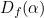we know that there is one minimum. We’ll find it by setting the derivative ofequal to 0. We have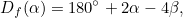where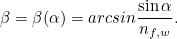To make things look simpler we’ll write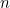for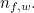Now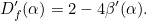The derivative of the function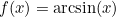is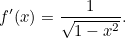Using the chain rule gives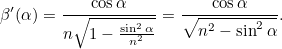Thus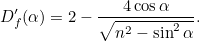Setting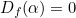gives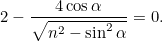Noting that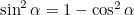and rearranging gives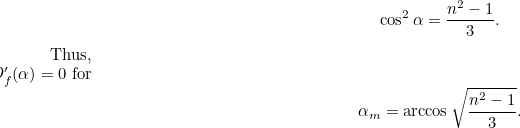Thus,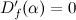for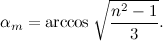Back to main article